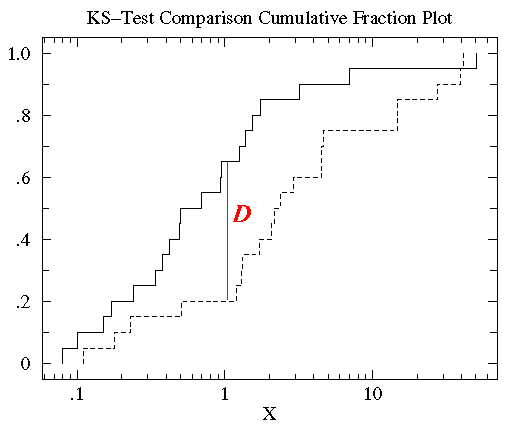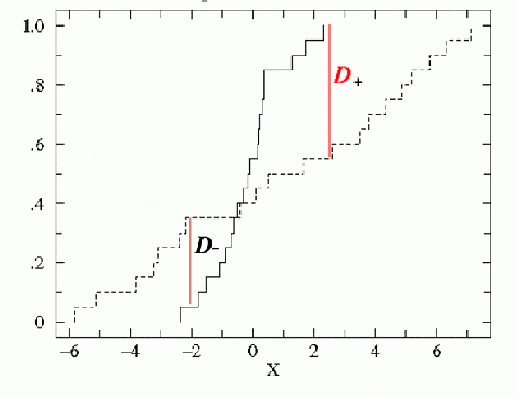## Summary

In the Chandra Source Catalog 2.0, a one-sample, two-sided Kolmogorov-Smirnov (K-S) test and a one-sample Kuiper's test are applied to the unbinned event data in each elliptical aperture that includes the 90% encircled counts fraction of the PSF to test the null hypothesis that the average intervals between arrival times of events are consistent with a constant count source rate throughout the observation. The null hypothesis is rejected if the value of the K-S statistic, D (defined below), is larger than a certain value. Corrections are made for good time intervals in the chip where each detection occurs, and for the source region dithering across regions of variable exposure during the observation. Note that background region information is not directly used in the K-S and Kuiper's variability tests in the Chandra Source Catalog, but is used in creating the Gregory-Loredo light curve for the background. The results of the K-S and Kuiper's variability tests are recorded in the columns ks_prob / kp_prob of the Source Observations Table and in the ks_intra_prob / kp_intra_prob of the Stacked Observations Detection Table and Master Sources Table.

### Dither correction

One of the ways by which telescope dither introduces variability into light curves is via modulation of the fractional area of a source region as it moves as a function of time over a chip edge/boundary, or as it moves as a function of time to chip regions with differing numbers of bad pixels or columns. The fractional area (including chip edge, bad pixel, and bad column effects) vs. time curves for source regions are calculated from the data, and are sufficient to correct the K-S and Kuiper's tests used in the Chandra Source Catalog for the effects of dither. This correction is implemented in the K-S/Kuiper's test model by integrating the product of the good time intervals with the fractional area vs. time curve; the cumulative integral of this product is the cumulative distribution function against which the data is compared. For further details, see the memo "Adding Dither Corrections to L3 Lightcurves".

Note that the dither correction described above is a geometrical area correction only that is applied to the data; it does not take into account any spatial dependence of the chip responses. For example, if a soft X-ray source dithers from a frontside-illuminated chip to a backside-illuminated chip, the different soft X-ray responses of the two chips could introduce a dither period-dependent modulation of the detected counts that is not accounted for simply by geometrical area changes. The current catalog procedures do not correct for such a possibility; however, warning flags are set if sources dither across chip edges, and a dither warning flag is set if the variability occurs at the harmonic of a dither frequency.

## Background

### Kolmogorov-Smirnov Test

The K-S test is a goodness-of-fit test used to assess the uniformity of a set of data distributions. It was designed in response to the shortcomings of the chi-squared test, which produces precise results for discrete, binned distributions only. The K-S test has the advantage of making no assumption about the binning of the data sets to be compared, removing the arbitrary nature and loss of information that accompanies the process of bin selection.

In statistics, the K-S test is the accepted test for measuring differences between continuous data sets (unbinned data distributions) that are a function of a single variable. This difference measure, the K-S $$D$$ statistic, is defined as the maximum value of the absolute difference between two cumulative distribution functions. The one-sided K-S test is used to compare a data set to a known cumulative distribution function, while the two-sided K-S test compares two different data sets. Each set of data gives a different cumulative distribution function, and its significance resides in its relation to the probability distribution from which the data set is drawn: the probability distribution function for a single independent variable $$x$$ is a function that assigns a probability to each value of $$x$$. The probability assumed by the specific value $$x_{i}$$ is the value of the probability distribution function at $$x_{i}$$ and is denoted $$P(x_{i})$$.The cumulative distribution function is defined as the function giving the fraction of data points to the left of a given value $$x_{i}$$, $$P(x <x_{i})$$; it represents the probability that $$x$$ is less than or equal to a specific value $$x_{i}$$.

Thus, for comparing two different cumulative distribution functions $$S_{N1}(x)$$ and $$S_{N2}(x)$$, the K-S statistic is

where $$S_{N}(x)$$ is the cumulative distribution function of the probability distribution from which a dataset with $$N$$ events is drawn. If $$N$$ ordered events are located at data points $$x_{i}$$, where $$i = 1, \ldots, N$$, then

where the $$x$$ data array is sorted in increasing order. This is a step function that increases by $$1/N$$ at the value of each ordered data point.###### Kirkman, T.W. (1996) Statistists to Use. http://www.physics.csbsju.edu/stats/

For our purposes, the two distributions compared are the measured distribution of arrival times, and the accumulated fraction on the time interval elapsed. If the null hypothesis (no variability) holds, we get 50% of your events in 50% of the passed time, and the $$D$$ statistic should distribute according to the Kolmogorov distribution for many realizations of the arrival times distribution. The probability returned by the test is thus $$p_{KS} = 1 - \alpha$$, where $$\alpha$$ is the probability (under the Kolmogorov distribution) that the value of $$D$$ is larger or equal than the measured value. A small value of $$p_{KS}$$ therefore indicates consistency with the null hypothesis, whereas a large value of $$p_{KS}$$ indicates that the interval between events is not constant, and therefore variability can be inferred.

### Kuiper's Test

While the K-S test is adept at finding shifts in a probability distribution, with the highest sensitivity around the median value, its power must be enhanced by other techniques to be as good at finding spreads, which affect the tails of a probability distribution more than the median value. One such technique is Kuiper's test, which compares two cumulative distribution functions via the Kuiper's statistic $$V$$, the sum of the maximum distance of $$S_{N1}(x)$$ above and below $$S_{N2}(x)$$:###### Kirkman, T.W. (1996) Statistists to Use. http://www.physics.csbsju.edu/stats/

If one changes the starting point of the integration of the two probability distributions, $$D_{+}$$ and $$D_{-}$$ change individually, but their sum is always constant. This general symmetry guarantees equal sensitivites at all values of $$x$$.